# Gasoline tank cylindrical

What is the inner diameter of the tank, which is 8 m long and contains 40 cubic cubic meters of gasoline?

D =  2.5231 m

### Step-by-step explanation: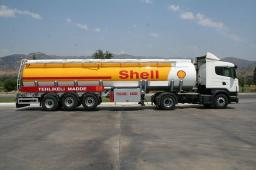Did you find an error or inaccuracy? Feel free to write us. Thank you!Tips to related online calculators
Tip: Our volume units converter will help you with the conversion of volume units.

## Related math problems and questions:

• Tank diameterA cylindrical tank has a volume of 60 hectoliters and is 2.5 meters deep. Calculate the tank diameter.
• A cylindrical tankA cylindrical tank can hold 44 cubic meters of water. If the radius of the tank is 3.5 meters, how high is the tank?
• Cylindrical tankCylindrical tank holds 600hl water and is deep 2.5 m. Calculate the diameter of the cylinder.
• Hectoliters of waterThere are 942 hectoliters of water in a cylindrical tank with an inner diameter of 6 m. The water reaches two thirds of the depth of the tank. Calculate its depth.
• Horizontal Cylindrical SegmentHow much fuel is in the horizontal cylindrical segment tank with a length of 10m, the width of level 1 meter, and the level is 0.2 meters below the tank's upper side?
• Diameter = heightThe cylinder's surface, the height of which is equal to the diameter of the base, is 4239 cm square. Calculate the cylinder volume.
• Cylindrical tank9.6 hl of water is poured into a cylindrical tank with a bottom diameter of 1.2 m. What height in centimeters does the water reach?
• CylinderIn a 1-meter diameter cylinder is 1413 liters of water, which is 60% of the cylinder. Calculate the cylinder height in meters, do not write the units. The resulting value round and write as an integer.
• Cylinder: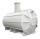Cylinder: calculate the height of a 3 liter cylindrical pot with a diameter of 10 cm.
• Pine woodFrom a trunk of pine 6m long and 35 cm in diameter with a carved beam with a cross-section in the shape of a square so that the square had the greatest content area. Calculate the length of the sides of a square. Calculate the volume in cubic meters of lu
• Top-open tank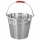The top-open tank has the shape of a truncated rotating cone, which stands on a smaller base. The tank's volume is 465 m3, the radii of the bases are 4 m and 3 m. Find the depth of the tank.
• The tankThe tank is full up to 4/5 of the total height and contains 240 hl of water. The area of the base is 6 square meters. What is the height of the tank?
• Fuel economyHow many kilometers is sufficient petrol in the cylinder fuel tank with a diameter of 40 cm and the base of tank length of 1 m, when it is filled to 60%, and if the car consumes 15 liters per 100 km?
• The solar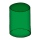The solar heating tank has the shape of a rotating cylinder. When the tank is in a horizontal position, the water in the tank wets 4/5 each tank bases. If we place the tank in a vertical position, the water in the tank will reach up to 1.2 m. Calculate th
• The volume 2The volume of a cube is 27 cubic meters. Find the height of the cube.
• The cylinderThe cylinder has a surface area of 300 square meters, while the cylinder's height is 12 m. Calculate the volume of this cylinder.
• Tank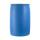In the middle of a cylindrical tank with a bottom diameter 251 cm is standing rod which is 13 cm above the water surface. If we bank rod its end reach surface of the water just by the tank wall. How deep is the tank?